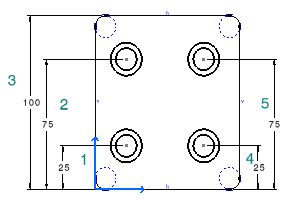# Setting Relations Between Dimensional ConstraintsThis task shows you how to set constraints between dimension using formulas. As a result, if you modify one of these dimensions (the driving dimension), all the other dimensions as well as the geometry will be modified accordingly.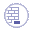Open the Brackets_views04.CATDrawing document.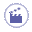1. Click the Formula icon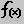in the Knowledge toolbar.
The Formulas dialog box appears.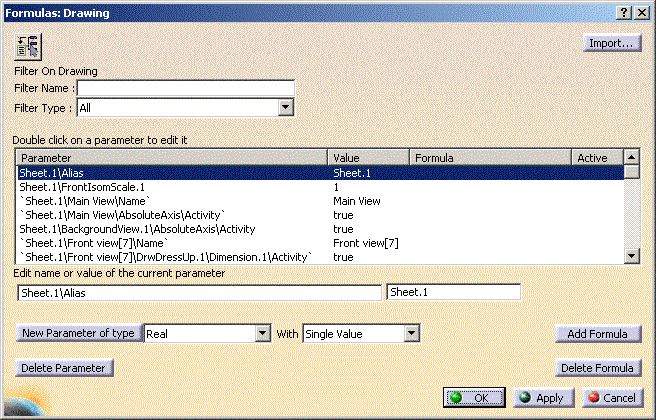You will now select, one after the other, the dimensions to be constrained and then enter in the dialog box the formulas to be used.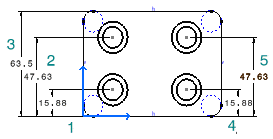2. Select a first dimension (1).

3. Click the Add Formula button in the Formulas dialog box.

The Formula Editor dialog box appears.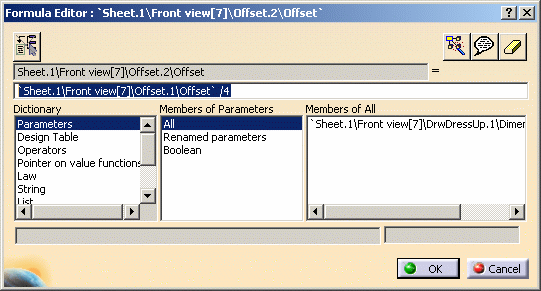4. Select a second dimension (3) and add "/4".

Then, click OK.

5. Back in the Formulas dialog box, select a first dimension (2).

6. Click the Add Formula button.

The Formula Editor dialog box appears.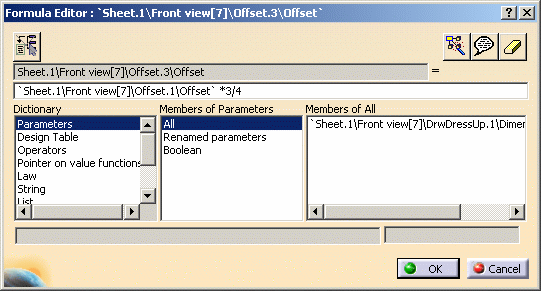7. Select a second dimension (3) and add "*3 /4". Then, click OK.

8. Back in the Formulas dialog box, select a first dimension (4).

9. Click the Add Formula button.

10. The Formula Editor dialog box appears.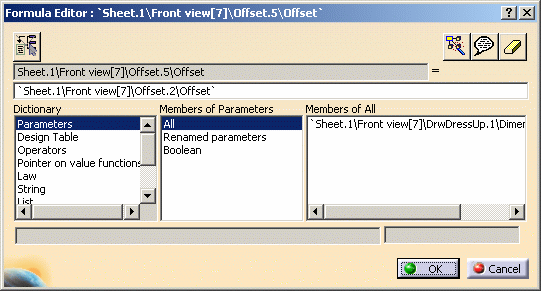11.  Select a second dimension (1) and then, click OK.

12. Back in the Formulas dialog box, select a first dimension (5).

13. Click the Add Formula button.

The Formula Editor dialog box appears.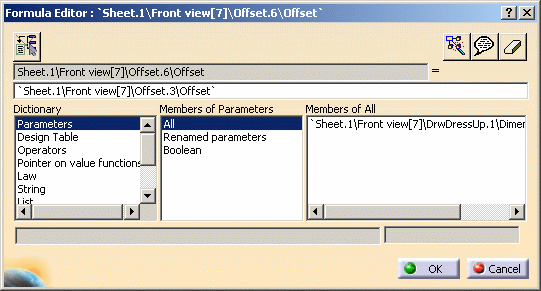14. Select a second dimension (2) and then, click OK.

15. Back in the Formulas dialog box, click OK.

Note that the specification tree is modified accordingly.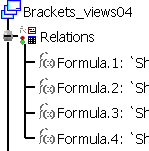16. Double-click the dimension to be set as driving dimension (3).

The Dimension Value dialog box appear.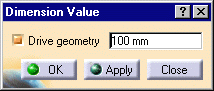17. If needed, activate the Drive geometry option.

18. Enter 100mm as dimension new length and press OK.

All the dimensions you previously constrained using formulas are automatically updated.